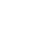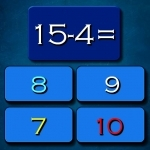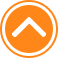×

PlaySubtraction Math Challenge70%Device: 💻 Pc   📱 Mobile
Rating: 70%
🎲Subtraction Math Challenge

Subtraction Math Challenge is fun math game suitable for all ages. I hope you like math and this game is just to test your math skills. Ten seconds to answer each quastion. Have fun playing. Here you can play Subtraction Math Challenge game and observe its walkthrough at no cost in kizi1000.com. Subtraction Math Challenge is an wonderful game from our list of Kizi 1000 games.

🎮 Instructions

Use Left Mouse button to Play

📂 Categories

puzzle, arcade, mathka, numbers, school, thinking, mathematical, mathematics, igrice, mathematic, math..

👌 Recommended
📌 Tags